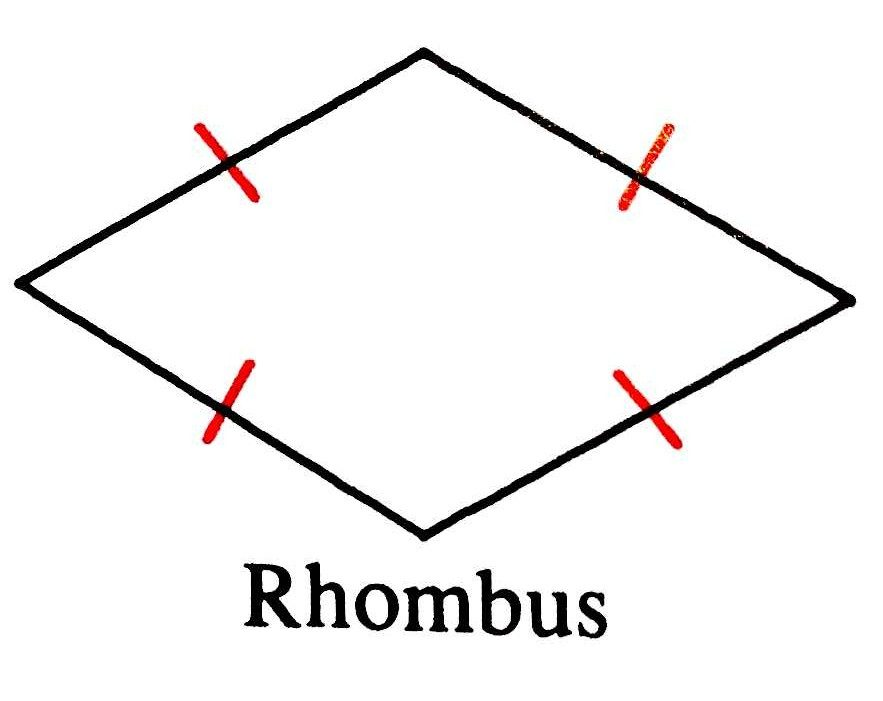# How to find the area of a rhombusA rhombus is the “jewel” of all parallelograms – after all, it is in the shape of a diamond. More technically, however, any shape that has four congruent sides is a rhombus. As you venture into the world of geometry, you will begin working with various shapes, including the rhombus. Getting to know more about it, its characteristics and how to work with it will help you along the way.

To feel confident you have found a rhombus, look for the following characteristics that all rhombuses possess. The rhombus is an equal opportunity parallelogram. No side is bigger or better than the other. In fact, they are all equal. The rhombus is part of the bigger group of parallelograms.

The altitude of any rhombus is the distance (at right angles) between two of the sides. When you create diagonal lines from each corner, they will bisect and create right angles.

The Area of a Rhombus

When you begin working with shapes in geometry, one of the first things you will do is find out all you can about it. One of these measurements is the area.

To calculate the area of a rhombus, you follow the steps here.

Step 1: Area = Altitude x s (s = side length)

Step 2: The length of the side squared, or multiplied by sine of angle A or B. This would be written as Area = sin(A).

Step 3: Multiply the diagonal lengths and then divide by 2. This would be written as Area = (p x q)/2.

For example, what if you were given a rhombus with diagonals of 8 m and 10 m. What would the area of this rhombus be? To solve this problem, you simply use the equation above.

Related text  How to relieve sore throat

Area = (8 m x 10 m)/2 =

The Perimeter of a Rhombus

The perimeter is defined as the distance around the edges of a rhombus. The perimeter is always equal to four times “s” (the length of the sides) since all of the sides are an equal length. This means that Perimeter = 4s.

For example, the side length of a rhombus is 10 cm, the perimeter would be 4(10) = 40.

Important Notes

Squares are rhombuses. This is just a rhombus that has all right angles. While the most common term used to describe this shape in geometry is “rhombus” you may also hear the shape referred to as a rhomb or diamond. The plural for several of these is rhombuses or rhombi. The actual word rhombus is derived from “rhombos” which is Greek and means a piece of wood that is whirled on a string to create a roaring noise.

Being able to work with a rhombus is essential. It will help you get through geometry and help you other times in life. Make sure to use the tips and equations here to ensure you can solve for the area and perimeter of any rhombus you are given.